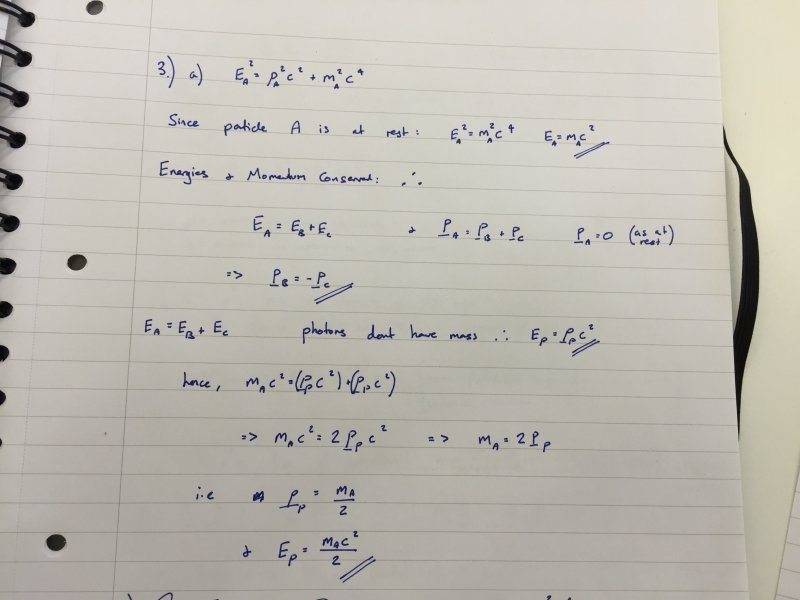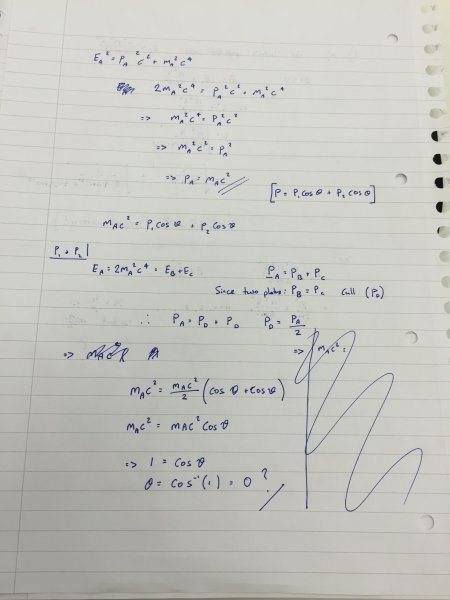# Angle of photon emission from particle decay (Particle Phys)

• 12x4

## Homework Statement

a) Particle A decays at rest into two photons. Calculate, in terms of the rest mass of A, the energy and momentum of each photon.

b) In a different reference frame, particle A is initially in motion such that its kinetic energy is equal to its rest energy. Find the momentum of A in this frame. Assuming that the photons have equal energy, use conservation of momentum to calculate the angle between the photons’ trajectories.

## Homework Equations

E2 = p2c2 + m2c4

EA = EB + EC

pA = pB + pC

p = p1 cosϑ + p2 cosϑ[/B]

## The Attempt at a Solution

:

A)[/B] PLEASE SEE THE ATTACHED PICTURE BELOW FOR MY WORKING

Okay so I think I have managed this part of the question and got an answer of:

photon momentum same for both photons and is : pp = mA / 2

Energy of each photon : Ep = mA c2 / 2B) PLEASE SEE THE ATTACHED PICTURE BELOW FOR MY WORKING

I am getting an angle of 0 degrees between the two photons. This is intuitively wrong so not sure what's happening. I'm going to try and upload an image of my working because it takes me ages to write it out on here and think it might be more clear what I've done. If it doesn't work then please bear with me and I'll write it out on here. thank you in advance to anyone that helps.

The second line of working i have just replaced the LHS with twice the rest mass.
Then followed that through to obtain a value for the momentum of A.

I then tried to use conservation of momentum to find a value for the momentum of each photon.

Once i had this i used the formula: p = p1 cosϑ + p2 cosϑIn part B you wrote ##P_A = P_B + P_C##. You need to treat the momenta as vectors. So, ##\vec{P}_A = \vec{P}_B + \vec{P}_C##. The right hand side is vector addition. This is in fact how you get to the relation involving the cosines of the angles.

What can you deduce about the photons from energy conservation?